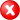#Boost C++ Libraries

...one of the most highly regarded and expertly designed C++ library projects in the world.

#### convert

Converts one geometry to another geometry.

###### Description

The convert algorithm converts one geometry, e.g. a BOX, to another geometry, e.g. a RING. This only works if it is possible and applicable. If the point-order is different, or the closure is different between two geometry types, it will be converted correctly by explicitly reversing the points or closing or opening the polygon rings.

###### Synopsis

```template<typename Geometry1, typename Geometry2>
void convert(Geometry1 const & geometry1, Geometry2 & geometry2)```

###### Parameters

Type

Concept

Name

Description

Geometry1 const &

Any type fulfilling a Geometry Concept

geometry1

A model of the specified concept (source)

Geometry2 &

Any type fulfilling a Geometry Concept

geometry2

A model of the specified concept (target)

Either

`#include <boost/geometry/geometry.hpp>`

Or

`#include <boost/geometry/algorithms/convert.hpp>`

###### Conformance

The function convert is not defined by OGC.

###### Supported geometries

Point

Segment

Box

Linestring

Ring

Polygon

MultiPoint

MultiLinestring

MultiPolygon

PointSegmentBoxLinestringRingPolygonMultiPointMultiLinestringMultiPolygonNoteIn this status matrix above: columns are source types and rows are target types. So a box can be converted to a ring, polygon or multi-polygon, but not vice versa.

Linear

###### Example

Shows how to convert a geometry into another geometry

```#include <iostream>

#include <boost/geometry.hpp>
#include <boost/geometry/geometries/box.hpp>
#include <boost/geometry/geometries/point_xy.hpp>
#include <boost/geometry/geometries/polygon.hpp>

BOOST_GEOMETRY_REGISTER_BOOST_TUPLE_CS(cs::cartesian)

int main()
{
typedef boost::geometry::model::d2::point_xy<double> point;
typedef boost::geometry::model::box<point> box;
typedef boost::geometry::model::polygon<point> polygon;

point p1(1, 1);
box bx = boost::geometry::make<box>(1, 1, 2, 2);

// Assign a box to a polygon (conversion box->poly)
polygon poly;
boost::geometry::convert(bx, poly);

// Convert a point to another point type (conversion of point-type)
boost::tuple<double, double> p2;
boost::geometry::convert(p1, p2); // source -> target

using boost::geometry::dsv;
std::cout
<< "box: " << dsv(bx) << std::endl
<< "polygon: " << dsv(poly) << std::endl
<< "point: " << dsv(p1) << std::endl
<< "point tuples: " << dsv(p2) << std::endl
;

return 0;
}
```

Output:

```box: ((1, 1), (2, 2))
polygon: (((1, 1), (1, 2), (2, 2), (2, 1), (1, 1)))
point: (1, 1)
point tuples: (1, 1)
```
Noteconvert is modelled as source -> target (where assign is modelled as target := source)